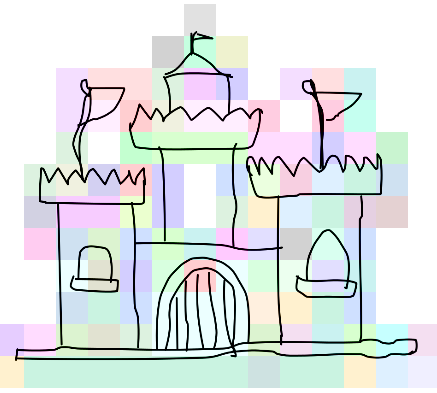### Algorithm Overview

Currently the goal of my project is to come up with essentially a clone of the program described in the paper for the project " How Do Humans Sketch Objects".

#### Classification Process

The classification process of a single image follows two steps. First, an image is converted from a graphical representation to a bag-of-words representation. Then a vector formed from the bag-of-words is categorized to an image group using support vector machines.

#### Feature Identification (Bag-of-Words)

Take a binary image of 256 x 256 pixels. First, compute the gradient of the image. This can be done efficiently by using the Sobel or the Scharr function. Convert the gradient into four discrete scalar fields (or images), where the magnitude of each scalar element is the magnitude of the vector at that point in either the x, y, -x, -y direction (or zero if the magnitude is negative.)

Create a bunch (784) of overlapping patches (90 x 90 pixels), and split each patch into 16 regions. For each region get four values, the sum of the magnitudes in each direction in that region, for a total of 64 values for each patch.Fig 1. A visualization of features in a sketch. Each feature in this example is 32x32 pixels; the image is sliced into 256 features and each one is colored based on its classification.

Now we have 784 64-dimensional feature vectors. Determine which of 500 clusters each vector belongs to (training data gives us 500 clusters using k-means clustering where k=500.) Now we have a feature for each feature vector, so we have 784 named features for our image.

#### Image Classification

Create a histogram from the 784 features by enumerating how many of each of the 500 features appear. Convert the histogram into a 500-dimensional vector. Classify this vector in a support-vector machine to get the image type.